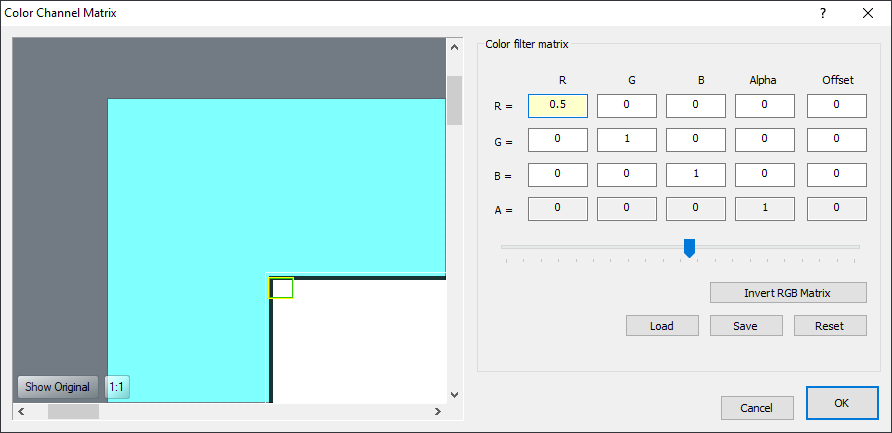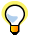...

### Chasys Draw IES Help:Effects : Custom via Color Channel Matrix

Introduction

The Color Channel Matrix is used to manipulate color and alpha values. It allows you to create saturation changes, hue rotation, luminance-to-alpha changes, and other color manipulation effects using values from one color channel and potentially applying them to other channels. The filter goes through the pixels in the source image one by one and separates each pixel into its red, green, blue, and alpha components. It then multiplies values provided in the color matrix by each of these values, adding the results together to determine the resulting color value that will replace that pixel.

The filter is controlled through a 5-by-4 matrix; illustrated below:The Math

As mentioned before, the filter is controlled through a 5-by-4 matrix. This is applied as follows:

 red = a[ 0] * srcR + a[ 1] * srcG + a[ 2] * srcB + a[ 3] * srcA + a[ 4] green = a[ 5] * srcR + a[ 6] * srcG + a[ 7] * srcB + a[ 8] * srcA + a[ 9] blue = a * srcR + a * srcG + a * srcB + a * srcA + a alpha = a * srcR + a * srcG + a * srcB + a * srcA + a

As you can see, the values in the first row determine the resulting red value, the second row the green value, the third row the blue value, and the fourth row the alpha value. You can also see that the values in the first four columns are multiplied with the source red, green, blue, and alpha values, respectively; while the fifth column value (offset) is added as is.Note that the source and result values for each channel range between 0 and 255. So even though the resulting value of red, green, or blue can be greater than 255 or less than 0, the values are constrained to this range.

Storage and Retrieval

The matrices can be stored to and retrieved from files; for this, one format is supported, a JpCHA2 Matrix format (.MAT) file containing a 5x4 matrix.